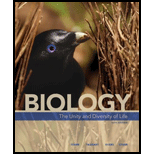# Refer to problem 2. Determine the predicted genotype frequencies among the offspring of the fol1owing crosses: a. AABB × aaBB b. AaBb × AABb c. AaBb × aa.bb d. AaBb × AaBb Question No: 2 2. Assuming that independent assortment occurs during meiosis, what type(s) of gametes will form in individuals with the following genotypes? a. AABB b. AaBB c. Aabb d. AaBb### Biology: The Unity and Diversity o...

15th Edition
Cecie Starr + 3 others
Publisher: Cengage Learning
ISBN: 9781337408332

#### Solutions

Chapter
Section### Biology: The Unity and Diversity o...

15th Edition
Cecie Starr + 3 others
Publisher: Cengage Learning
ISBN: 9781337408332
Chapter 13, Problem 3GP
Textbook Problem
255 views

## Refer to problem 2. Determine the predicted genotype frequencies among the offspring of the fol1owing crosses: a. AABB × aaBB b. AaBb × AABb c. AaBb × aa.bb d. AaBb × AaBb Question No: 22. Assuming that independent assortment occurs during meiosis, what type(s) of gametes will form in individuals with the following genotypes? a. AABB b. AaBB c. Aabb d. AaBb

a.

Summary Introduction

To predict: The predicted genotype frequencies among the offspring for the dihybrid cross AABB × aaBB.

Introduction: The law of independent assortment and law of segregation are the laws proposed by Gregor Johann Mendel, a geneticist. Mendel proposed the law of independent assortment based on the results of monohybrid cross that “two alleles at any locus tend to separate from each other during meiosis, so they end up in different gametes”. Mendel proposed the law of independent assortment based on the results of dihybrid cross that “alleles at one locus tend to assort into gametes independently of alleles at other loci”. The number of possible allele combination for the given genotype is given by the formula 2n where “n” is the number of heterozygous alleles that are present.

### Explanation of Solution

A monohybrid cross is constructed with Punnett square for the parents having the genotype AABB and aaBB...

b.

Summary Introduction

To predict: The predicted genotype frequencies among the offspring for the dihybrid cross AaBB × AABb.

Introduction: The law of independent assortment and law of segregation are the laws proposed by Gregor Johann Mendel, a geneticist. Mendel proposed the law of independent assortment based on the results of monohybrid cross that “two alleles at any locus tend to separate from each other during meiosis, so they end up in different gametes”. Mendel proposed the law of independent assortment based on the results of dihybrid cross that “alleles at one locus tend to assort into gametes independently of alleles at other loci”. The number of possible allele combination for the given genotype is given by the formula 2n where “n” is the number of heterozygous alleles that are present.

c.

Summary Introduction

To predict: The predicted genotype frequencies among the offspring for the dihybrid cross AaBb × aabb.

Introduction: The law of independent assortment and law of segregation are the laws proposed by Gregor Johann Mendel, a geneticist. Mendel proposed the law of independent assortment based on the results of monohybrid cross that “two alleles at any locus tend to separate from each other during meiosis, so they end up in different gametes”. Mendel proposed the law of independent assortment based on the results of dihybrid cross that “alleles at one locus tend to assort into gametes independently of alleles at other loci”. The number of possible allele combination for the given genotype is given by the formula 2n where “n” is the number of heterozygous alleles that are present.

d.

Summary Introduction

To predict: The predicted genotype frequencies among the offspring for the dihybrid cross AaBb × AaBb.

Introduction: The law of independent assortment and law of segregation are the laws proposed by Gregor Johann Mendel, a geneticist. Mendel proposed the law of independent assortment based on the results of monohybrid cross that “two alleles at any locus tend to separate from each other during meiosis, so they end up in different gametes”. Mendel proposed the law of independent assortment based on the results of dihybrid cross that “alleles at one locus tend to assort into gametes independently of alleles at other loci”. The number of possible allele combination for the given genotype is given by the formula 2n where “n” is the number of heterozygous alleles that are present.

### Still sussing out bartleby?

Check out a sample textbook solution.

See a sample solution

#### The Solution to Your Study Problems

Bartleby provides explanations to thousands of textbook problems written by our experts, many with advanced degrees!

Get Started

Find more solutions based on key concepts
Athletes should avoid frequent between-meal snacks. T F

Nutrition: Concepts and Controversies - Standalone book (MindTap Course List)

Distinguish between acids and bases. What are their properties?

Biology: The Dynamic Science (MindTap Course List)

30-80 Does the debranching enzyme help in digesting amylose?

Introduction to General, Organic and Biochemistry

Complementary foods can be introduced 3 months. __________ True _______False

Nutrition Through the Life Cycle (MindTap Course List)

Referring to Figure CQ28.6, describe what happens to the lightbulb after the switch is closed. Assume the capac...

Physics for Scientists and Engineers, Technology Update (No access codes included)

What is a high-energy compound?

Organic And Biological Chemistry

How does basin shape influence tidal activity?

Oceanography: An Invitation To Marine Science, Loose-leaf Versin